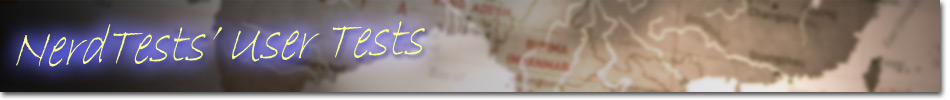Commonly Misspelled Words

This test will help improve your achievement test scores. It is a multiple choice spelling test of commonly misspelled words.

1)

Choose the correct spelling from the three choices given.

2)

Choose the correct spelling from the three choices given.

3)

Choose the correct spelling from the three choices given.

4)
 Choose the correct spelling from the three choices given.   accumulate   accummulate   acummulate
5)

Choose the correct spelling from the three choices given.

6)

Choose the correct spelling from the three choices given.

7)

Choose the correct spelling from the three choices given.

8)

Choose the correct spelling from the three choices given.

9)

Choose the correct spelling from the three choices given.The word is defined as: (noun) Direction given to another to help.

10)

Choose the correct spelling from the three choices given.defined as: A (verb) to give direction to another individual to help.

11)

Choose the correct spelling from the three choices given.

12)

Choose the correct spelling from the three choices given.

13)

Choose the correct spelling from the three choices given.

14)

Choose the correct spelling from the three choices given.

15)

Choose the correct spelling from the three choices given.

16)

Choose the correct spelling from the three choices given.

17)

Choose the correct spelling from the three choices given.

18)

Choose the correct spelling from the three choices given.

19)

Choose the correct spelling from the three choices given.

20)

Choose the correct spelling from the three choices given.